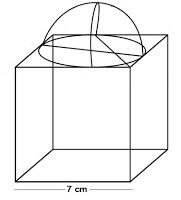# A cubical block of side 7 cm is surmounted by a hemisphere. What is the greatest diameter the hemisphere can have? Find the surface area of the solid.each side of cube is 7 cm.

So, the radius = 7/2 cm.

Surface area of solid=T.S.A of cube-Area of circle+ C.S.A of hemisphere

=6*side*side-πr2+2πr2

=6*side*side+πr2

=6*7*7+22/7*7/2*7/2

=294+72/2

=294+38.5

=332.5cm2